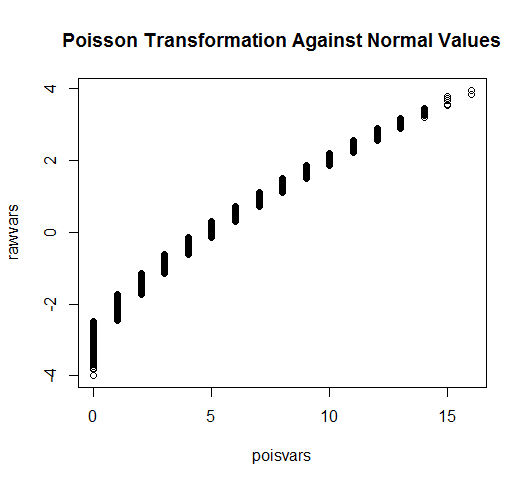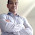## Thursday, February 27, 2014

### Easily generate correlated variables from any distribution

In this post I will demonstrate in R how to draw correlated random variables from any distribution

The idea is simple.
1. Draw any number of variables from a joint normal distribution. 2. Apply the univariate normal CDF of variables to derive probabilities for each variable. 3. Finally apply the inverse CDF of any distribution to simulate draws from that distribution.
The results is that the final variables are correlated in a similar manner to that of the original variables.  This is because the rank order of the variables in maintained and thus correlations are approximately the same though not exact. This methods follows a method I presented in a previous post coded in Stata.  I am not aware of anybody else proposing this method previously.

For example:
```library(MASS)

# We will use the command mvrnorm to draw a matrix of variables

# Let's keep it simple,
mu <- rep(0,4)
Sigma <- matrix(.7, nrow=4, ncol=4) + diag(4)*.3

rawvars <- mvrnorm(n=10000, mu=mu, Sigma=Sigma)

cov(rawvars); cor(rawvars)
# We can see our normal sample produces results very similar to our ```
```#specified covariance levels.

# No lets transform some variables
pvars <- pnorm(rawvars)

# Through this process we already have
cov(pvars); cor(pvars)
# We can see that while the covariances have dropped significantly, ```
```# the simply correlations are largely the same.

plot(rawvars[,1], pvars[,2], main="Normal of Var 1 with probabilities of Var 2") ```
` `
`# Things are looking pretty well so far.  Let's see what happens when we invert `
```#different CDFs.

# To generate correlated poisson
poisvars <- qpois(pvars, 5)
cor(poisvars, rawvars)
# This matrix presents the correlation between the original values generated
# and the tranformed poisson variables.  We can see that the correlation matrix
# is very similar though somewhat "downward biased".  This is because any
# transformation away from the original will reduce the correlation between the
# variables.

plot(poisvars,rawvars, main="Poisson Transformation Against Normal Values")```
` `
```# Perhaps the poisson count distribution is not exotic enough.

# Perhaps a binomial distribution with 3 draws at 25% each
binomvars <- qpois(1-pvars, 3, .25)
# Note, I did 1-p because p is defined differently for the qpois for some ```
```#reason
cor(binomvars, rawvars)

# Or the exponential distribution
expvars <- qexp(pvars)
cor(expvars, rawvars)
# We can see that the correlations after the exponential tranformations are
# significantly weaker (from .7 to .63) but still good representations if
# we are interested in simulating correlations between a normal and exponential
# variables.

plot(expvars,rawvars, main="Exponential Transformation Against Normal Values") ```

```# To make things a little more interesting, let's now transform our probabilities
# into skewed normal distributions.
library(sn)
sknormvars <- qsn(pvars, 5, 2, 5)
cor(expvars, rawvars)

hist(sknormvars, breaks=20) ```
```# Finally in order to demonstrate what we can do let's combine our variables into
# a single matrix.

combvar <- dataframe(sknormvars[,1], poisvars[,2], binomvars[,3], expvars[,4])

cor(combvar)
#          [,1]      [,2]      [,3]      [,4]
#[1,] 1.0000000 0.6853314 0.6826398 0.6256086
#[2,] 0.6853314 1.0000000 0.6748458 0.6402233
#[3,] 0.6826398 0.6748458 1.0000000 0.6325102
#[4,] 0.6256086 0.6402233 0.6325102 1.0000000

# I am going to try to get all of these dirstributions on the same graph
stdcombvar <- t(t(combvar)-apply(combvar,2,min))
stdcombvar <- t(t(stdcombvar)/apply(stdcombvar,2,max))
summary(stdcombvar)

plotter <- data.frame(
values = c(stdcombvar),
rawnorm = rep(rawvars[,1], 4),
type = rep(c("skewed normal",
"poisson",
"binomial",
"exponential"),
each=10000))

library(ggplot2)  ```
```
ggplot(plotter, aes(x=rawnorm ,y=values, color=type)) +
geom_point(shape=1) +     # Use hollow circles
geom_smooth(method=lm,    # Add linear regression line
se=FALSE)  ```
` `

` `
Formatted by Pretty R at inside-R.org

1.Thank you for this! I have been trying to build this functionality in R through the "datasynthR" package. https://github.com/jknowles/datasynthR I think I have implemented this method in many cases, but not all. I will be referring to this post as I continue to expand the package.

2.Cool post. I think the method has been around for a while under multiple names (adds to confusion). Here are some references.
http://onlinelibrary.wiley.com/doi/10.1002/asmb.901/pdf

I think the method is very much related to the probability integral transform.
http://en.wikipedia.org/wiki/Probability_integral_transform

Would be cool to know more details regarding the pros/cons of this method versus copulas. And if any other general purpose methods exist for generating correlated data?

1.Thanks for these citations. That is very helpful here is the MLA citations:

Cario, Marne C., and Barry L. Nelson. Modeling and generating random vectors with arbitrary marginal distributions and correlation matrix. Technical Report, Department of Industrial Engineering and Management Sciences, Northwestern University, Evanston, Illinois, 1997.

Yahav, Inbal, and Galit Shmueli. "On generating multivariate poisson data in management science applications." Robert H. Smith School Research Paper No. RHS (2009): 06-085.

3.Hi Francis,

I enjoyed your R demo on Thursday related to generating correlated random variables. However, it seems to me that applying "pnorm" without specifying the means via which to center the multivariate normal variates won't yield exactly uniform random variates, and therefore the output of the q- functions won't come from the specified distributions. It may come from some more generalized non-central version however, but that I won’t pretend to understand.

I also do not understand why you write "without copulas”. If I'm right about the bug and it's corrected, at that point the routine implements first 1) simulation of a Gaussian copula via the mvnorm and pnorm steps (due to the probability integral transform of normal marginals of a multivariate normal) and 2) simulation of the desired marginals using their q-functions transformation to the desired marginals. Though I’m no copula specialist, I thought this was exactly how you use a Gaussian copula to generate correlated random variables with specified marginals. For example, this is discussed under the heading “Monte Carlo integration for copula models” on the Wikipedia page for copulas. Perhaps the term “copula” intimidates some readers, but I think it’d be better to "take ownership of it” (to use an idiom of political activists) rather than avoid it. The idea is to link the technical mathematical edifice to a more nuts and bolts understanding of how someone might use a copula. That way we get any benefits of the theoretical edifice and bring that to a wider audience.

In case it helps, some recent statistics literature refers to joining estimates of Pearson correlations and arbitrary marginals as estimation of a non-paranormal distribution.
http://repository.cmu.edu/cgi/viewcontent.cgi?article=2024&context=compsci

Best wishes,
Bryce

1.Great points Bryce especially with regards to the copula question. Apparently, I was using some form of Copulas. As for your first point I think you may be mistaken. The distribution of the multivariate normals each are defined as mean 0 and variance 1. I only modified the non-diagnol portion of the variance/covariance matrix. Therefore, using pnorm without specifying mean and variance correctly transforms the normal variables into uniform variables.

Observe:
> rawvars <- mvrnorm(n=100000, mu=mu, Sigma=Sigma)
> var(rawvars)
[,1] [,2] [,3] [,4]
[1,] 1.0011314 0.7044411 0.7034198 0.7007685
[2,] 0.7044411 1.0092061 0.7068980 0.7068707
[3,] 0.7034198 0.7068980 1.0062027 0.7051700
[4,] 0.7007685 0.7068707 0.7051700 1.0039373

> summary(rawvars)
V1 V2 V3 V4
Min. :-4.115731 Min. :-4.512754 Min. :-4.219649 Min. :-3.976831
1st Qu.:-0.679732 1st Qu.:-0.681546 1st Qu.:-0.679985 1st Qu.:-0.680782
Median :-0.005315 Median :-0.002374 Median :-0.002781 Median :-0.001728
Mean :-0.004668 Mean :-0.001369 Mean :-0.003259 Mean :-0.004019
3rd Qu.: 0.675674 3rd Qu.: 0.683425 3rd Qu.: 0.676256 3rd Qu.: 0.673854
Max. : 4.712489 Max. : 4.336386 Max. : 5.606908 Max. : 4.630360

4.Hi Francis,

Thanks for bringing this to our attention.

The NORTA procedure (from normal, through uniform, to anything) works very well for us, because it preserves the Spearman rank correlations:

1. For the normal and the uniform variables, the Pearson correlations are related by rho_normal = 2 sin (rho_uniform x pi/6),

2. For the uniform variables, the Pearson and Spearman correlations are identical,

3. So for the final variables, the Spearman correlation can be specified without any error (unless there is probability mass at particular points).

By the way, I do not agree with you that the NORTA is “without copulas”. They are still there, in the intermediate step, but they can be ignored if you want to.

Wilbert

5.This comment has been removed by the author.